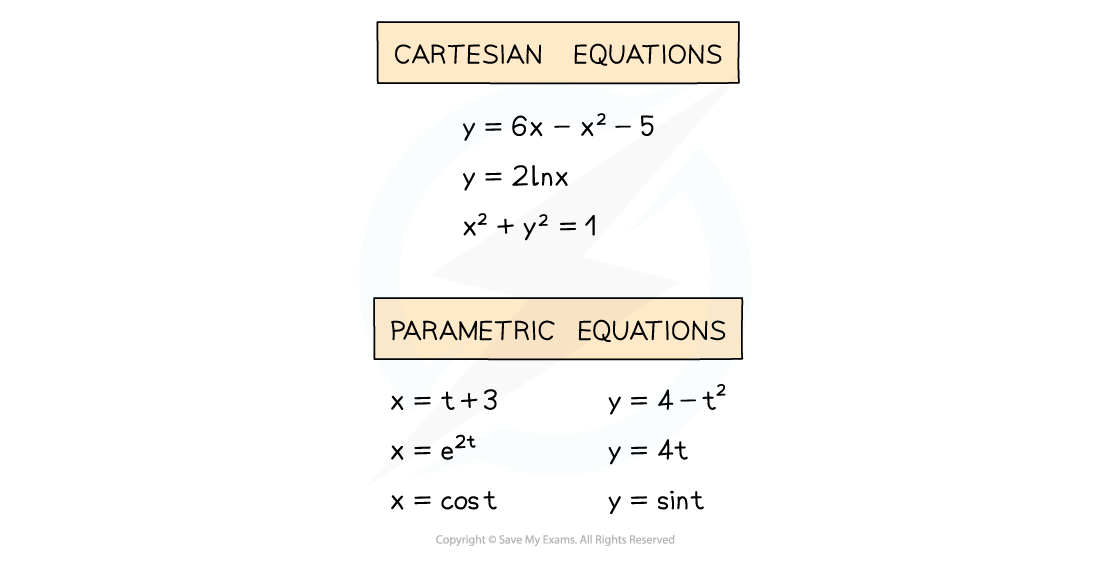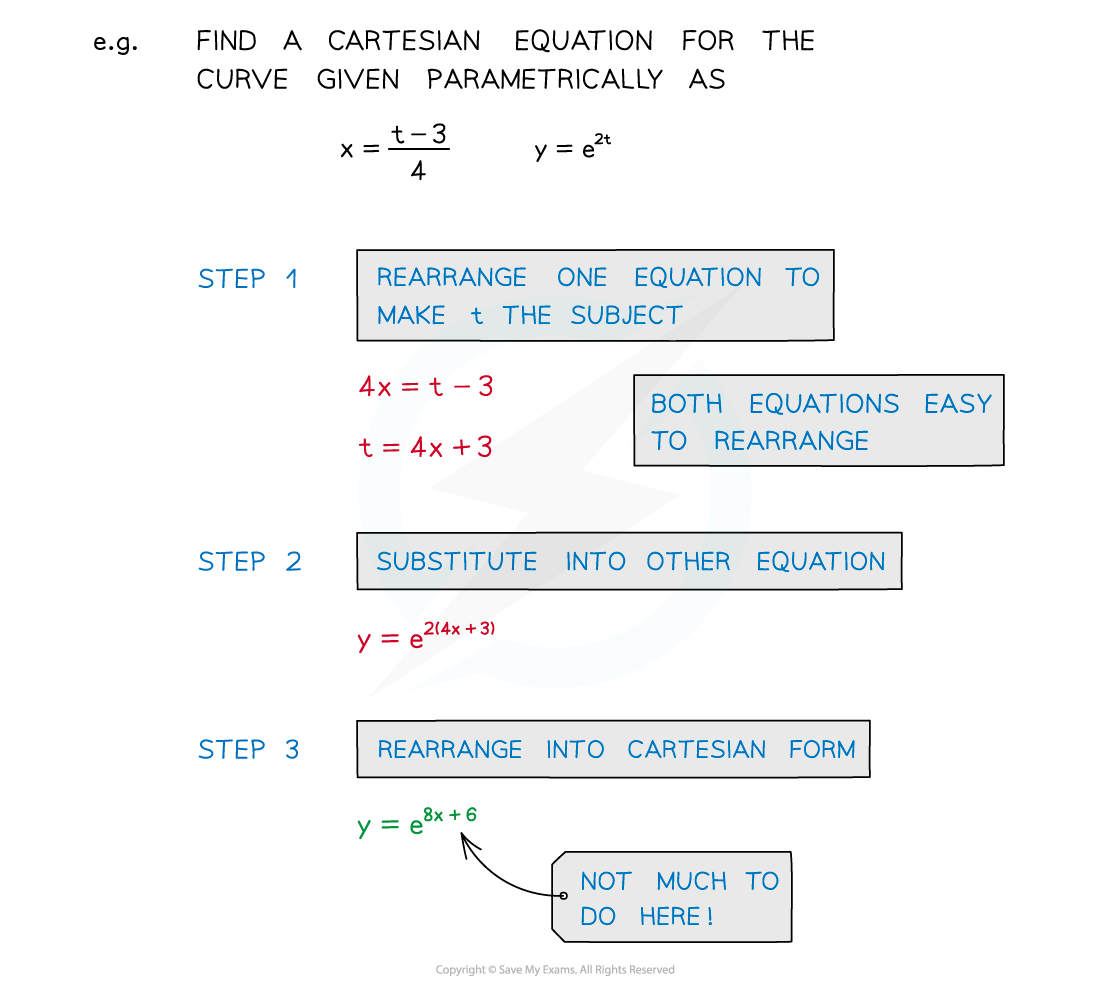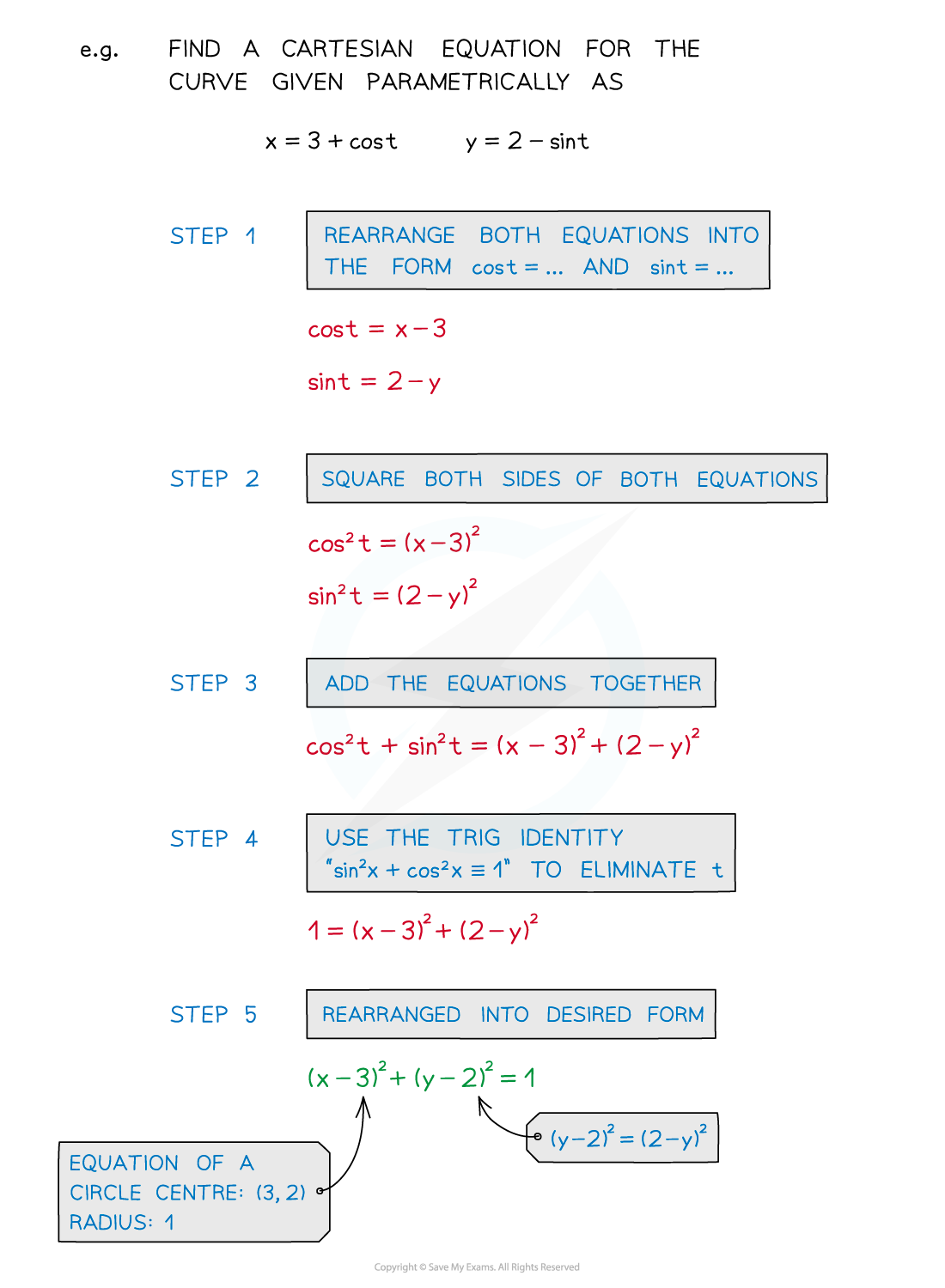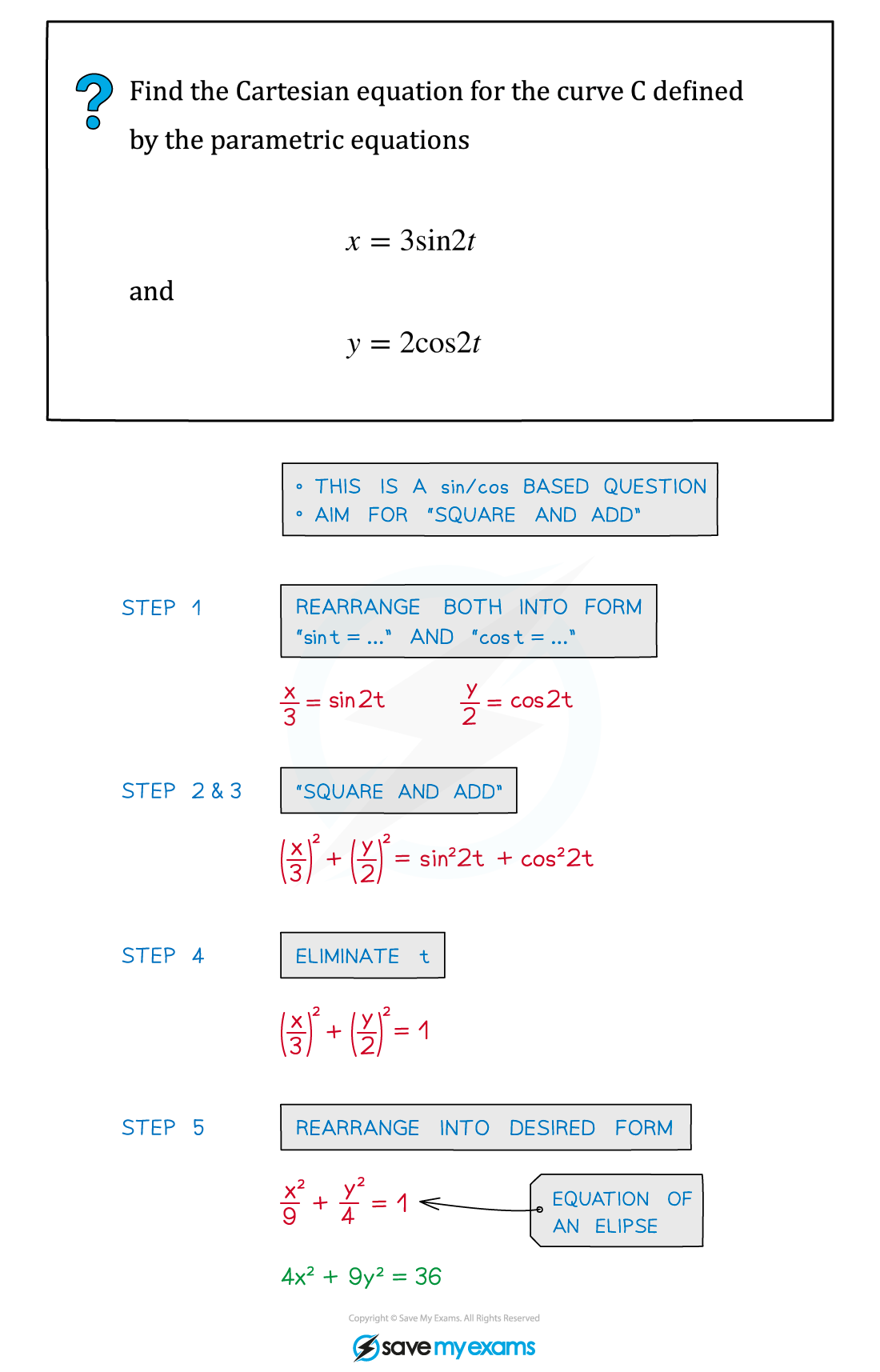# AQA A Level Maths: Pure复习笔记9.1.2 Parametric Equations - Eliminating the Parameter

### Parametric Equations - Eliminating the Parameter

#### What does eliminating the parameter mean?• In parametric equations, x = f(t) and y = g(t)
• There is still a connection directly linking x and y
• This will be the Cartesian equation of the graph

#### How do I find the Cartesian equation from parametric equations?• STEP 1: Rearrange one of the equations to make t the subject
• Either t = p(x) or t = q(y)
• STEP 2: Substitute into the other equation
• STEP 3 Rearrange into the desired (Cartesian) form

#### How do I eliminate t when trig is involved?• STEP 1  Rearrange both equations into the forms “cos t = …” and “sin t = …”
• STEP 2  Square BOTH sides of BOTH equations
• STEP 3  Add the equations together
• STEP 4  The trig identity “sin2 x + cos2 x ≡ 1” eliminates t
• STEP 5  Rearrange into desired (Cartesian) form
• This technique is seen in Trigonometric Identities

#### Exam Tip

When choosing which equation to rearrange, aim for “as simple as possible”:

• Linear equations are simpler than quadratics
• eg Rearrange x = 2t + 3or

y = 3t2 +3t -4 ?

• Single exponential terms are quite easy to deal with
• eg x = et → t = ln x

Trig identities may be needed and remember squared terms are good!

• eg sin2 x + cos2 x ≡ 1

#### Worked Example